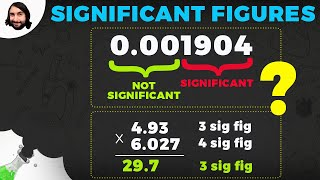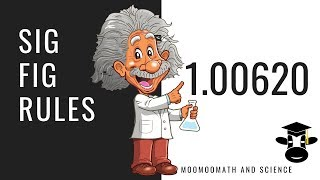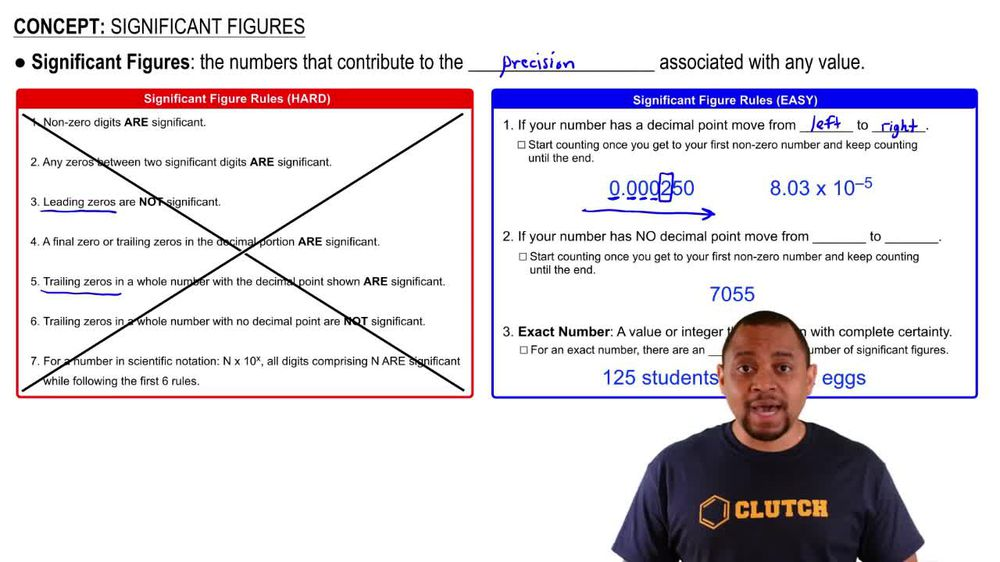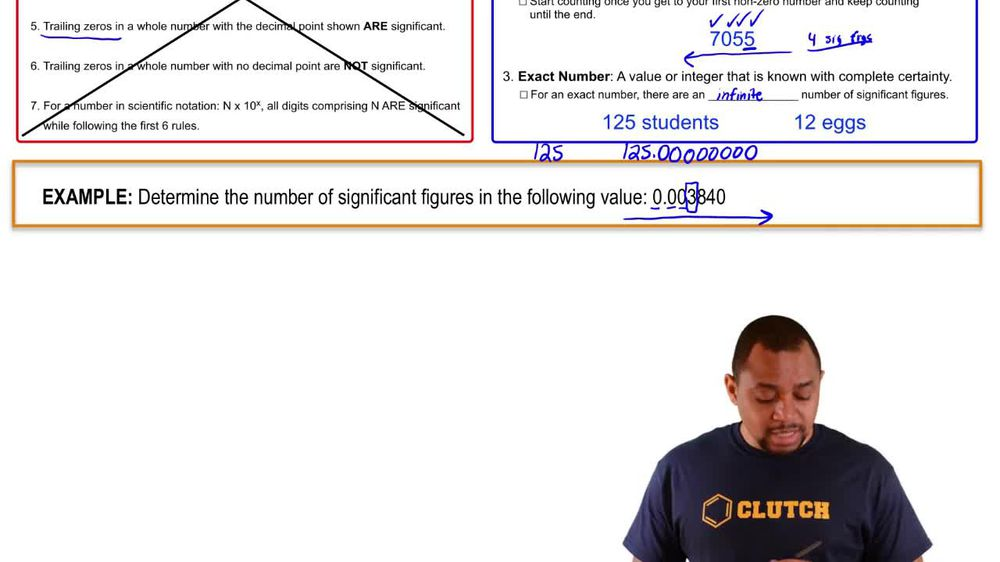Start typing, then use the up and down arrows to select an option from the list.
1. 1. Intro to General Chemistry2. Significant Figures
Problem

# Which of the following statements uses exact numbers? (a) 1 ft = 12 in. (b) 1 cal = 4.184 J (c) The height of Mt. Everest is 29,035 ft. (d) The world record for the 1-mile run, set by Morocco’s Hicham el Guerrouj in July 1999, is 3 minutes, 43.13 seconds

Relevant Solution1m
Play a video:
Hello everyone today we are being given the falling problem identify the statements that use exact numbers. A says the height of the Eiffel Tower from base to tip is m. This is a result from measuring so it's a result from measurement and therefore this is not exact so it's not exact. So answer choice A is not the answer and so answer choice is A. And E can be removed. B says one inch equals 2.54 centimeters. By definition this is an exact number. So be checks out. So answer choice D. Is wrong. She says the current world record for the 100 m dash is 9.58 seconds set by Jamaica's Usain bolt in 2009. Once again as was in a this is a result from measurement. So this was a measurement taken and therefore it is not exact. So any answer traces with C. Are going to be incorrect. And this leaves Andrew choice C. B. And D. As the answer just for completeness, let's look at D one U. S. Liquid court is equal to 32 US fluid ounces. This is an example of an exact number. So be into your correct answer choice C. Is correct. And we have answered the question. I hope this helped and until next time